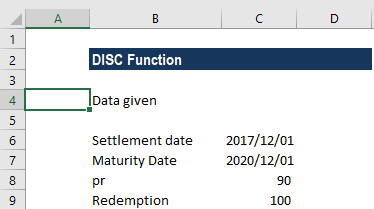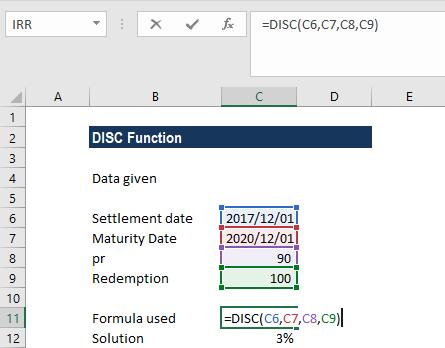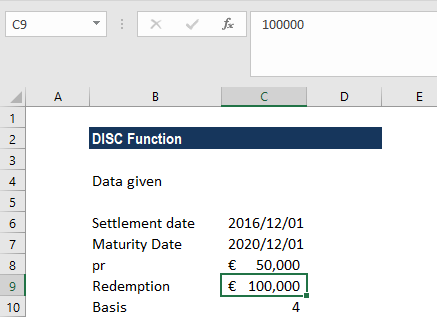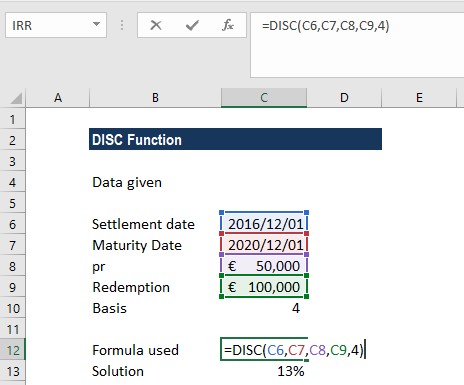# DISC Function

Calculates the rate of discount for a bond

## What is the DISC Function?

The DISC Function is an Excel Financial function. It will calculate the rate of discount for a bond. It helps to know the discount rate when we know the other details about the bond.

### Formula

=DISC(settlement, maturity, pr, redemption, [basis])

The DISC function uses the following arguments:

1. Settlement (required argument) – This is the date when the coupon was purchased. It is the security’s settlement date or the date after the issue date when the security is traded to the buyer.
2. Maturity (required argument) – This is the maturity date of the security or the date on which the security expires.
3. Pr (required argument) – The security’s price per \$100 face value.
4. Redemption (required argument) – This is the security’s redemption value per \$100 face value.
5. Basis (optional argument) – Defines the day count basis that is used in the calculation. The possible values can be as follows:

BasisDay Count basis
0 or omittedUS(NASD) 30/360
1Actual/actual
2Actual/360
3Actual/365
4European 30/360

### How to use the DISC Function in Excel?

To understand the uses of the DISC function, let’s consider a few examples:

#### Example 1

Suppose we are given the following data:The formula to use is:We get a result of 3%. If the result is in number format, we need to convert it into percentage format.

In the example above:

1. We omitted the basis argument so the function took it as 0, which denotes the US (NADS) 30/360 day count basis).
2. We gave the date arguments to the DISC function as references to cells containing dates. If the date format is not used, we will get an error as a result.

#### Example 2

Let’s take another example. Suppose we are given information about a European bond:The formula to use is:We get a result of 13%. In the example:

1. We used the basis 4 so the function took it as 4, which denotes the European 30/360 count basis.
2. We gave the date arguments to the DISC function as references to cells containing dates. Remember, based on the settings of your system, text representations of dates in Excel functions may vary.

### Things to remember about the DISC Function

• #NUM! error – Occurs when:
• The given maturity date is less than or equal to the settlement date.
• Inputs for the arguments pr, redemption or basis are invalid numeric values. That is, if pr is less than or equal to 0, if redemption is less than or equal to 0, or basis given in the formula is not equal to 1, 2, 3, or 4.
• Settlement, maturity, and basis are truncated to integers.
• Dates are stored in sequential serial numbers, so they can be used in calculations. By default, January 1, 1900 is serial number 1, and July 16, 2016 is serial number 42567 because it is 42,567 days after January 1, 1900.
• #VALUE! error – Occurs when:
• The given maturity date or settlement date is not a valid Excel date.
• Any of the given argument is a non-numeric value.

Thanks for reading CFI’s guide to the Excel DISC function. By taking the time to learn and master these Excel functions, you’ll significantly speed up your financial analysis. To learn more, check out these additional CFI resources:

• Excel Functions for Finance